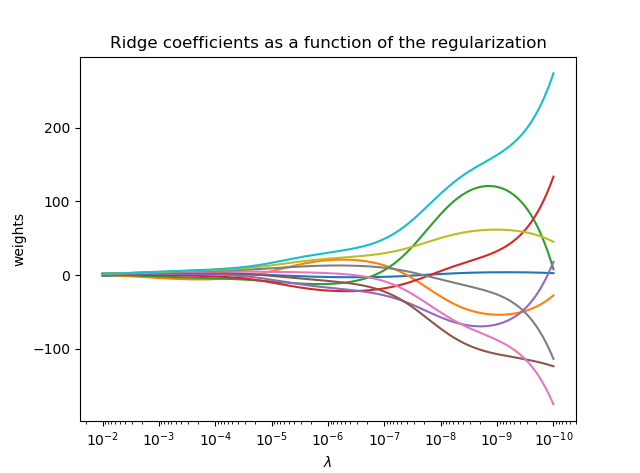#### 怎样解决过拟合#### 岭回归的原理2.其次构建惩罚函数，针对不同的λ，画出岭迹图。
3.根据岭迹图，选择要剔除那些特征。

#### 一个sckit-learn的example

import numpy as np
import matplotlib.pyplot as plt
from sklearn import linear_model

#X为一个10*10的矩阵
X = 1. / (np.arange(1, 11) + np.arange(0, 10)[:, np.newaxis])
y = np.ones(10)

##############################################################################
#设置不同的lambda和参数

n_lambda = 200
lambda = np.logspace(-10, -2, n_lambda)

coefs = []
for a in lambda:
ridge = linear_model.Ridge(lambda=a, fit_intercept=False)
ridge.fit(X, y)
coefs.append(ridge.coef_)

# #############################################################################
#显示绘制结果

ax = plt.gca()

ax.plot(lambda, coefs)
ax.set_xscale('log')
ax.set_xlim(ax.get_xlim()[::-1])  # reverse axis
plt.xlabel(r'$\lambda$')
plt.ylabel('weights')
plt.title('Ridge coefficients as a function of the regularization')
plt.axis('tight')
plt.show()